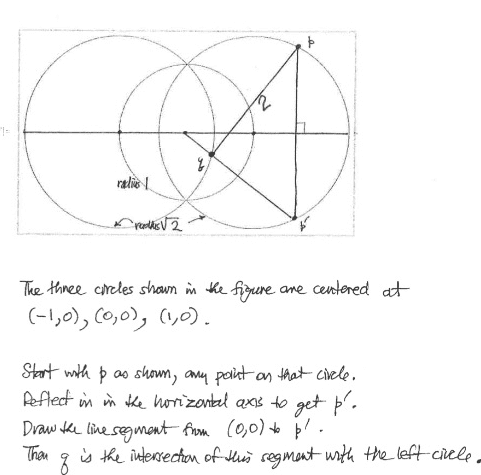# Geometry proof

Mod note: Member warned that homework questions must be posted in the Homework & Coursework sections
http://imgur.com/zGB2dnY

Was given this problem a few weeks ago and I'm not sure how I should be approaching it. Please let me know which theorems I should look into in order to solve the problem.

Last edited by a moderator:

As I can see the the circle with radius 1 and centered on origin seems tobe redundant. Then why is it given. may be that is some hint. I am also trying to solve. for p(√2,0) the required distance can be easily proved = 2. Tr it then try to solve the general problem.

As I can see the the circle with radius 1 and centered on origin seems tobe redundant. Then why is it given. may be that is some hint. I am also trying to solve. for p(√2,0) the required distance can be easily proved = 2. Tr it then try to solve the general problem.

Ok I figured it out but the middle circle actually does play a crucial role. So first the center of the left circle to the radius at the right is sqrt(2). Subtract 1 to find the space in between the origin and that spot on the right. If we set that point on the right of the left circle to be point q we can use it. From q to the center of the right has to be .59 because you subtract .41 from 1. Then if we set b to be (sqrt2,0) we know from the right center to the point is sqrt2. Add the .59 to it and you get two.

I think you are referring to the situation when p and p' coincide or respectively coincide with intersection points of the circles. For those special cases we can easily prove that the distance is 2 and for the latter case the extra circle helps. But the general problem is still unsolved for me.

http://imgur.com/zGB2dnY

Was given this problem a few weeks ago and I'm not sure how I should be approaching it. Please let me know which theorems I should look into in order to solve the problem.
Drawing is not at scale.

SammyS
Staff Emeritus
Homework Helper
Gold Member
Drawing is not at scale.
It looks reasonably close to scale to me.•theBin
It looks reasonably close to scale to me.
View attachment 100324
Sorry, now I see that it is at scale. I guess it could be proven by analytic geometry combined with (infinitesimal) calculus. High school geometry in the plan, permits only to make a series of tests for different positions of the points p, p' and q;

I think it has to do with construction of two triangles with sides √2 and 1 but the angle is not included one.

I have understood the significance of the circle at the origin of radius 1. That immediately tells us that the angle which PP' subtends at origin is twice the angle which qq' subtends at center (-1, 0) of the first circle. This can help us to prove the result.

Let point (-1. 0) be labelled as a and (1, 0) as b and (0, 0) as o. Consider the triangles: qbp and qba. qb side is common and bp = qa = √2 (given). So if we can prove the included angle ∠qbp = ∠aqb, then the triangles can be proved to be congruent and thus pq = ab = 2 given.